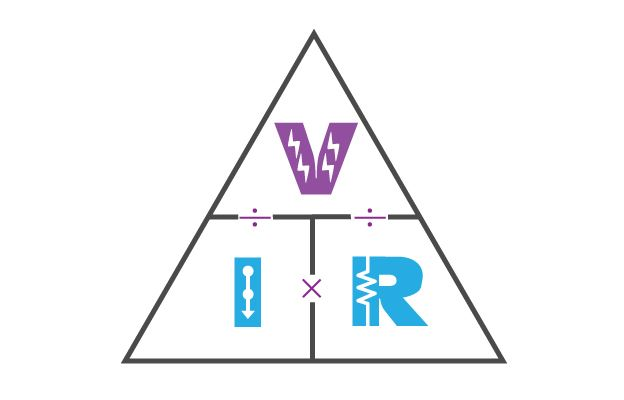# Ohm’s Law

According to Ohm’s Law that the amount of current in a electrical circuit is directly proportional to the voltage. Ohm expressed his discovery in the form of a simple equation, describing how voltage, current, and resistance interrelate:

In this algebraic expression, voltage (E) is equal to current (I) multiplied by resistance (R). Using algebra techniques, we can manipulate this equation into two variations, solving for I and for R, respectively:

### Analyzing Simple Circuit

Let’s see how these equations might work to help us analyze simple circuits:

Moreover, In the above circuit, there is one source of voltage (battery) and one source of resistance (the lamp). This makes it very easy to apply Ohm’s Law. If we have given any two of the values we can calculate the third one.

we will calculate the amount of current (I) in a circuit, given values of voltage (E) and resistance (R): Below is the example.

What is the amount of current (I) in this circuit?

Now we have the values of Current and Voltage so we have to find the Resistance. Find the below example.

What is the amount of resistance (R) offered by the lamp?

### Limitations of Ohm’s Law

Following are the limitations of Ohm’s law:

1. it is not applicable for unilateral electrical elements like diodes and transistors as they allow the current to flow through in one direction only. Therefore ohm law is not applicable on it.
2. For non-linear electrical elements with parameters like capacitance, resistance etc the voltage and current won’t be constant with respect to time making it difficult to use Ohm’s law.

### The Ohms law Triangle

If any of the two values provide while the third value is not provided then we can find it by the below mention formula.## One Comment to “Ohm’s Law”

1.Ntl

very goooood